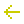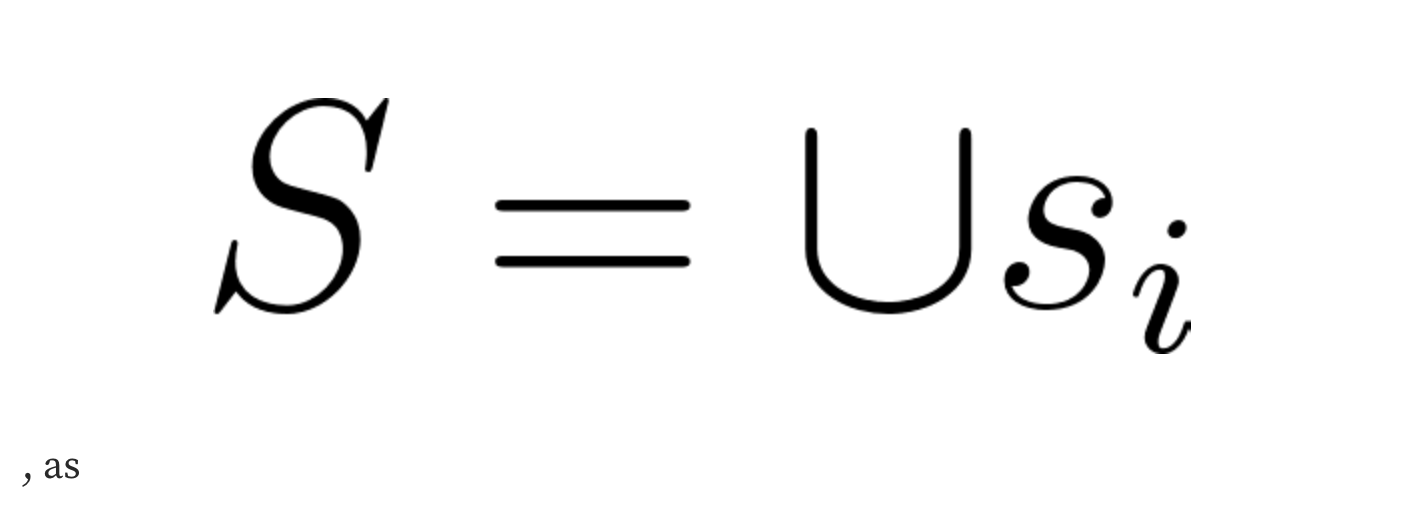Y2K Blog# Introducing Earthquake PT 2Earthquake is our flagship structured product that allows users to hedge, speculate and underwrite the volatility risk associated with various pegged assets. Users can take on these positions by depositing \$ETH in fully collateralized insurance vaults that leverage a variant of the ERC 4626 token standard. This second article will cover the payout calculations and the pricing model for the vaults.

# Payout Calculations

Here is the methodology via which Payouts are calculated for the hedge and risk.

We define the following variables:

- Sj is the collateral posted by each insurance seller
- NB is the number of insurance buyers
- NS is the number of insurance sellers, and
- c is the trading fee taken by the protocol.

In the event of no depegging event- in addition to the return of their collateral.

If there is a depegging event,- Each seller j net paysNumerical Example

Let B1 = 1, B2 = 3, S1 = 150, S2 = 200, S3 = 50, c = 0.005. It is clear that, before accounting for trading fees, the yield in this scenario for a single epoch is 1%.

In the event of no depegging event,

- Buyer 1 pays 1 ETH,
- Buyer 2 pays 3 ETH,
- Seller 1 receives 1.5 × 99.5% ETH
- Seller 2 receives 2 × 99.5% ETH, and
- Seller 3 receives 0.5 × 99.5% ETH

If there is a depegging event

- Buyer 1 pays 1 ETH and receives 100 ETH, leaving him with (100 × 99.5% − 1) ETH,
- Buyer 2 pays 3 ETH and receives 300 ETH, leaving him with (300 × 99.5% − 3) ETH,
- Seller 1 receives 1.5 ETH and pays 150 ETH, leaving him with (−150 + 1.5 × 99.5%) ETH,
- Seller 2 receives 2 ETH and pays 200 ETH, leaving him with (−200 + 2 × 99.5%) ETH, and
- Seller 3 receives 0.5 ETH and pays 50 ETH, leaving him with (−50+ 0.5× 99.5%) ETH.

Determining Strike Prices

Y2K Finance aims to eventually expand to provide insurance markets for various different stablecoin protocols. Here we provide a sample methodology for determining strike prices, which someone may seek to use when creating new Y2K vaults. At genesis, Y2K epochs will be assigned on a per-month basis; that is to say, there will be an August epoch, September epoch, etc. This is subject to change according to protocol governance votes.

The methodology presented below is for a stablecoin pegged to 1 USD. In this framework, we assign three strikes to each stablecoin:

- K1 is the riskiest strike, which is expected to be breached every 3 months.
- K2 is a “medium risk” strike, which is defined as being breached every 18 months.
- K3 is a “low risk” strike, which denotes black swan events. These are the lowest yielding but provide protection against events that are unexpected to ever occur over the lifetime of a stablecoin.

We assume that price deviations from \$1 are independent and identically distributed random variables.Where Si is the stable coin price at a given time Ti. It is well known that in times of mass decollateralization spirals, these variables become correlated and the i.i.d. the assumption above does not hold. We deal with this by assuming that the discrete time series of stablecoin prices is sampled from a “continuous” (block-by-block) series

It is well known that in times of mass decollateralization spirals, these variables become correlated and the i.i.d. the assumption above does not hold. We deal with this by assuming that the discrete time series of stablecoin prices is sampled from a “continuous” (block-by-block) series Ŝ,Where we assume that any correlation spirals happen within each intervalEach strike Kk∈{1,2,3}has an associated rate rk , which is defined as the probability that the strike is breached within a given ΔT . The rate is calculated using an indicator function from the discrete time seriesand can be used in a binomial distribution to find the probability Pk of a particular strike being breached within a given month:Where f is the sampling frequency and d is the number of days in a given epoch. The equation above is then solved for each rk

on the intervalgiven the desired values of Pk. This can be done using a variety of root finding algorithms. Once

rk is determined, the set of all Xi can be iterated through for varying strikes until an appropriate K is found. For the cases K1 and K2 , each Pk, respectively, is 1/3 and 1/18

This wraps up our series covering Earthquake’s mechanics, simulations for the protocol’s pricing models can be found in the Whitepaper. You can follow our Twitter account @Y2KFinance and join our discord for updates and announcements.

Discord: https://discord.gg/tvuBNSW3